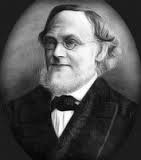# The Weak and Strong Goldbach ConjecturesIn 1742 the German mathematician Christian Goldbach wrote a letter to Leonhard Euler proposing two problems that, until recently, have seen little progress.  In modern terms, the problems are identified as either the Weak or Strong Goldbach Conjecture.  The Strong Goldbach Conjecture is the statement that every even integer greater than 2 is the sum of two prime numbers.  Similarly, the modern version of the Weak conjecture can be stated as every odd integer greater than 5 is the sum of three odd primes.

In his groundbreaking work earlier this year, the Peruvian mathematician Harald Helfgott (with much acceptance in the math community) announced that he had proved the Weak conjecture.  Fascinated by his result, I sent him an email of congratulations with a related question involving the Strong conjecture.  The following was his reply:

Dear Avery,

Thank you for your email. The fact that “three implies four” (so to speak)
was already known (and, as you can see, very easy to prove). I believe the
strong conjecture is much, much, much harder.

All the best
Harald Helfgott

I too agree that the Strong conjecture is, “much,much,much harder”.      On May 13, 2013, the renowned mathematician Terrence Tao of UCLA released the following statement on the internet in regards to Helfgott’s result:

Busy day in analytic number theory; Harald Helfgott has complemented his previous paper http://arxiv.org/abs/1205.5252 (obtaining minor arc estimates for the odd Goldbach problem) with major arc estimates, thus finally obtaining an unconditional proof of the odd Goldbach conjecture that every odd number greater than five is the sum of three primes….. As with virtually all successful partial results on the Goldbach problem, the argument proceeds by the Hardy-Littlewood-Vinogradov circle method; the challenge is to make all the estimates completely effective and to optimise all parameters (which, among other things, requires a certain amount of computer-assisted computation). [EDIT: the proof also relies on extensive numerical verifications of GRH that were performed by David Platt.]”

Unfortunately, Tao further stated that it would be unlikely that the Hardy-Littlewood Vinogradov Circle Method and Helfgott’s result could be used to prove the Strong conjecture.  Therefore the mystery of the Strong conjecture has currently escaped all attempts of proof.    Computationally, it has been shown true for every even integer greater than 2 into the trillions.   It would be clever indeed  for some mathematician to show that the Strong conjecture follows trivially from the Weak.  Do you think you can do it?Avery Carr is a senior analyst and past senior editor for the American Mathematical Society Grad Blog. He and his wife, Alison, live in Olive Branch, MS.
This entry was posted in AMS, General, Math, Math in Pop Culture, News, Uncategorized. Bookmark the permalink.

### 5 Responses to The Weak and Strong Goldbach Conjectures

1.john hebert says:

About goldbach’s conjecture, what about the primes 2 plus 5 equaling 7, an odd number? Doesn’t this example discount the gold Bach conjecture?

•Aneesh Nirmalan says:

2+3+2

•Pranit Verma says:

Goldbach conjecture state that every even integer greater than two is the sum of two prime numbers. It doesn’t specify us about the sum of two prime number to be odd. It perfectly state that the even integer greater than two are the sum of two prime number.

2.Jenaro Tomaszewski says:

An even stronger version of the conjecture is that every even number greater than 6 is the sum of two distinct primes

3.Mans Nilsson says:

John Hebert, what you’re mentioning is uninteresting, as it can easily be shown to be false in general over the odd numbers, i.e. 11 is not a Goldbach number as 9 is not a prime number. In fact, any odd number is a Goldbach number iff the odd integer that is two less than that number is a prime number (an odd number plus an odd number is an even number, so one of the primes must be even, i.e. 2).